Author: Oscar Cronquist Article last updated on December 03, 2021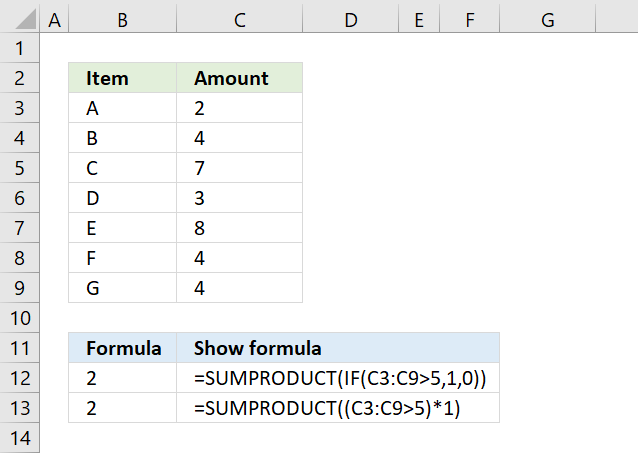You don't need to use the IF function in a SUMPRODUCT function, it is enough to use a logical expression. For example, the array formula above in cell B12 counts all cells in C3:C9 that are above 5 using an IF function.

## 1. How to simplify IF functions in the SUMPRODUCT function=SUMPRODUCT(IF(C3:C9>5,1,0))

The first argument in the IF function is a logical expression, use that in your SUMPRODUCT formula. The formula in B13 does the same thing as in B12.

=SUMPRODUCT((C3:C9>5)*1)

You need to tell Excel the order of calculation, in other words, make sure you calculate C3:C9>5 before you multiply by 1. To do that use parentheses.

(C3:C9>5)*1

The larger than comparison operator compares each value in C3:C9 if larger than 5. It returns an array the same size as C3:C9 containing boolean values, TRUE or FALSE.

{FALSE; FALSE; TRUE; FALSE; TRUE; FALSE; FALSE}*1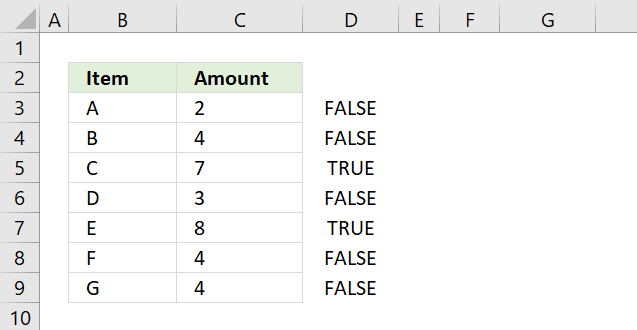The picture above shows the array in column D, value 7 and 8 are larger than 5.

The SUMPRODUCT function can't sum boolean values so multiply (using the asterisk *) the logical expression by 1 to convert the boolean values (TRUE and FALSE) to numbers (1 or 0).

{0;0;1;0;1;0;0}

The asterisk also has another advantage, you can enter the SUMPRODUCT formula as a regular formula.

### 1.1 Combine SUMPRODUCT and IF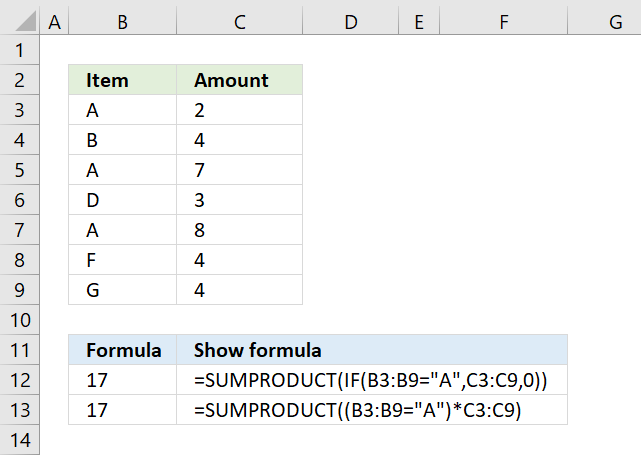The picture above demonstrates an IF function that checks if a condition is met and if TRUE returns a value in a corresponding cell.

There is no need for the IF function in the above example, simply use the logical expression and multiply with the values you want to use, demonstrated in cell B13.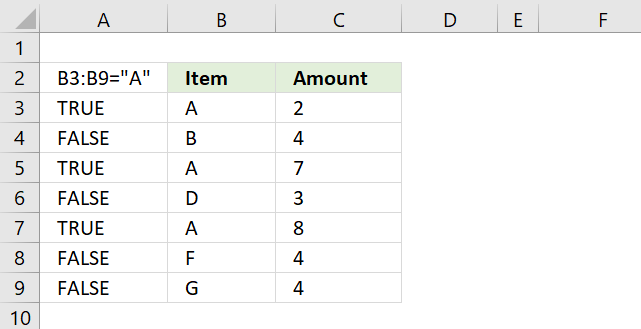B3:B9="A" returns {TRUE;FALSE;TRUE;FALSE;TRUE;FALSE;FALSE} displayed in column A in image above.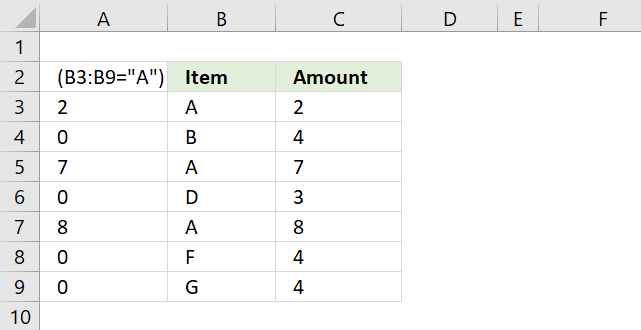(B3:B9="A")*C3:C9 returns this array: {2;0;7;0;8;0;0} shown in column A.Lastly, the SUMPRODUCT function sums all the numbers in the array returning 17 in cell B12.

2 + 7 + 8 = 17.

## 2. SUMPRODUCT if greater than 0 (zero)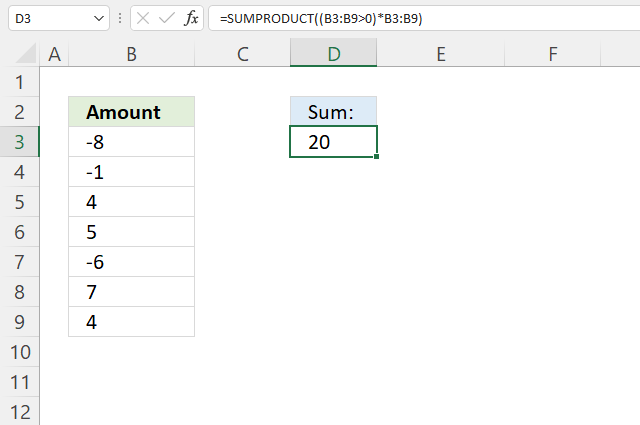The formula in cell D3 adds numbers from B3:B9 if they are larger than 0 (zero) and returns a total.

Formula in cell D3:

=SUMPRODUCT((B3:B9>0)*B3:B9)

### 2.1 Explaining formula

#### Step 1 - Check if values are larger than 0 (zero)

The larger than sign is a boolean operator that compares values to a condition, it returns TRUE or FALSE based on the outcome.

B3:B9>0

becomes

{-8; -1; 4; 5; -6; 7; 4}>0

and returns

{FALSE; FALSE; TRUE; TRUE; FALSE; TRUE; TRUE}.

#### Step 2 - Multiply boolean values with numbers

The parentheses let you control the order of calculation, we must do the comparisons before we multiply.

(B3:B9>0)*B3:B9

The asterisk character lets you multiply a value with another value, in this case, with a boolean value.

(B3:B9>0)*B3:B9

becomes

{FALSE; FALSE; TRUE; TRUE; FALSE; TRUE; TRUE}*B3:B9

TRUE is equal to 1 and FALSE is equal to 0 (zero), this will create the following outcomes:

TRUE * number = number
FALSE * number = 0 (zero)

{FALSE; FALSE; TRUE; TRUE; FALSE; TRUE; TRUE}*B3:B9

becomes

{FALSE; FALSE; TRUE; TRUE; FALSE; TRUE; TRUE}*{-8; -1; 4; 5; -6; 7; 4}

and returns {0; 0; 4; 5; 0; 7; 4}.

Notice how all the negative numbers are converted to 0's (zeros).

The SUMPRODUCT function calculates the product of corresponding values and then returns the sum of each multiplication.

SUMPRODUCT(array1, [array2], ...)

SUMPRODUCT((B3:B9>0)*B3:B9)

becomes

SUMPRODUCT({0; 0; 4; 5; 0; 7; 4})

and returns 20 in cell D3.## 3. SUMPRODUCT if between two dates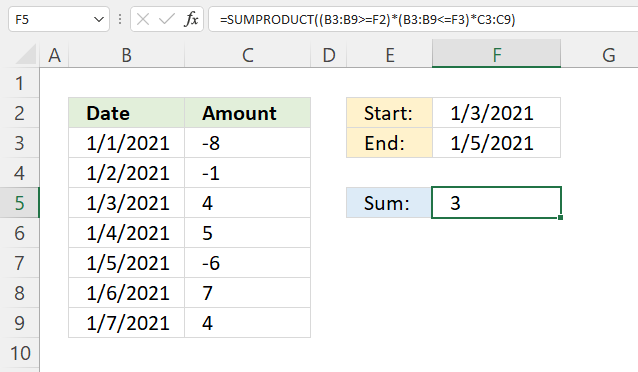The SUMPRODUCT function shown in cell F5 calculates a total based on two dates. The example above demonstrates the start date in F2 and end date in F3, cells B5, B6, and B7 have dates that match the date range.

The corresponding numbers are in cells C5, C6, and C7. The total is calculated like this 4 + 5 - 6 equals 3.

Formula in cell f5:

=SUMPRODUCT((B3:B9>=F2)*(B3:B9<=F3)*C3:C9)

### 3.1 Explaining formula

#### Step 1 - Check which dates are equal to or later than the start date

The larger than and the equal signs are boolean operators that compare values to a condition, it returns TRUE or FALSE based on the outcome.

B3:B9>=F2

becomes

{44197; 44198; 44199; 44200; 44201; 44202; 44203}>=44199

and returns

{FALSE; FALSE; TRUE; TRUE; TRUE; TRUE; TRUE}.

#### Step 2 - Check which dates are equal to or earlier than the end date

The less than and the equal signs are boolean operators that compare values to a condition, it returns TRUE or FALSE based on the outcome.

B3:B9<=F3

becomes

{44197; 44198; 44199; 44200; 44201; 44202; 44203}<=44201

and returns

{TRUE; TRUE; TRUE; TRUE; TRUE; FALSE; FALSE}.

#### Step 3 - Multiply arrays

The asterisk character lets you multiply values, this works fine with boolean values as well. This means that you can apply AND logic between the arrays.

TRUE*TRUE = 1
TRUE*FALSE = 0 (zero)
FALSE*TRUE= 0 (zero)
FALSE*FALSE= 0 (zero)

(B3:B9>=F2)*(B3:B9<=F3)

becomes

{FALSE; FALSE; TRUE; TRUE; TRUE; TRUE; TRUE}*{TRUE; TRUE; TRUE; TRUE; TRUE; FALSE; FALSE}

and returns {0; 0; 1; 1; 1; 0; 0}.

#### Step 4 - Multiply array with numbers

(B3:B9>=F2)*(B3:B9<=F3)*C3:C9

becomes

{0; 0; 1; 1; 1; 0; 0}*C3:C9

becomes

{0; 0; 1; 1; 1; 0; 0}*{-8; -1; 4; 5; -6; 7; 4}

and returns {0; 0; 4; 5; -6; 0; 0}.

#### Step 5 - Add numbers and return a total

The SUMPRODUCT function calculates the product of corresponding values and then returns the sum of each multiplication.

SUMPRODUCT(array1, [array2], ...)

SUMPRODUCT((B3:B9>=F2)*(B3:B9<=F3)*C3:C9)

becomes

SUMPRODUCT({0; 0; 4; 5; -6; 0; 0})

and returns 3 in cell F5. 4 + 5 - 6 = 3

## 4. SUMPRODUCT if cell contains text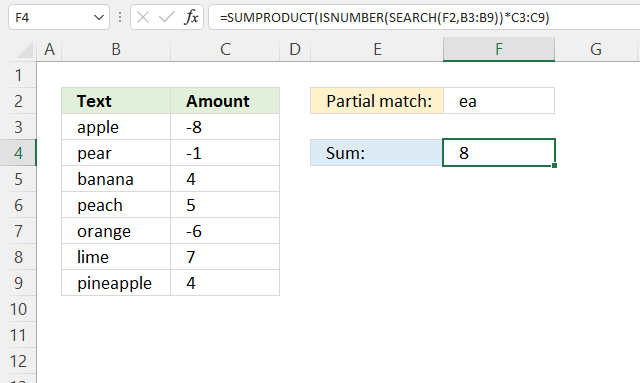The SUMPRODUCT function displayed in cell F4 calculates a total based on a string specified in cell F2. If a cell in B3:B9 contains the string (partial match) the corresponding number in cell C3:C9 is added to a total.

The image above demonstrates that the string is found in cells B4, B6, and B9. The corresponding cells in column C are C4, C6, and C9. The total is calculated like this: -1 + 5 + 4 equals 8.

Formula in cell F4:

=SUMPRODUCT(ISNUMBER(SEARCH(F2, B3:B9))*C3:C9)

### 4.1 Explaining formula

#### Step 1 - Find cells containing the string

The SEARCH function returns a number representing the position of character at which a specific text string is found reading left to right. It is not a case-sensitive search.

SEARCH(find_text,within_text, [start_num])

SEARCH(F2, B3:B9)

becomes

SEARCH("ea",{"apple";"pear";"banana";"peach";"orange";"lime";"pineapple"})

and returns {#VALUE!; 2; #VALUE!; 2; #VALUE!; #VALUE!; 4}.

The SEARCH function returns an error value if the string is not found.

#### Step 2 - Convert result to boolean values

The error values break the formula, we need to take care of possible error values.

The ISNUMBER function checks if a value is a number, returns TRUE or FALSE. This works fine with error values as well.

ISNUMBER(value)

ISNUMBER(SEARCH(F2, B3:B9))

becomes

ISNUMBER({#VALUE!; 2; #VALUE!; 2; #VALUE!; #VALUE!; 4})

and returns {FALSE; TRUE; FALSE; TRUE; FALSE; FALSE; TRUE}.

Notice that the error values are now boolean value FALSE.

#### Step 3 - Multiply with numbers

Boolean values can be used in multiplication, TRUE has the numerical equivalent of 1 and FALSE is 0 (zero).

TRUE * number = number
FALSE * number = 0 (zero)

ISNUMBER(SEARCH(F2, B3:B9))*C3:C9

becomes

{FALSE; TRUE; FALSE; TRUE; FALSE; FALSE; TRUE}* {-8; -1; 4; 5; -6; 7; 4}

and returns {0; -1; 0; 5; 0; 0; 4}.

SUMPRODUCT(ISNUMBER(SEARCH(F2, B3:B9))*C3:C9)

## 5. SUMPRODUCT if or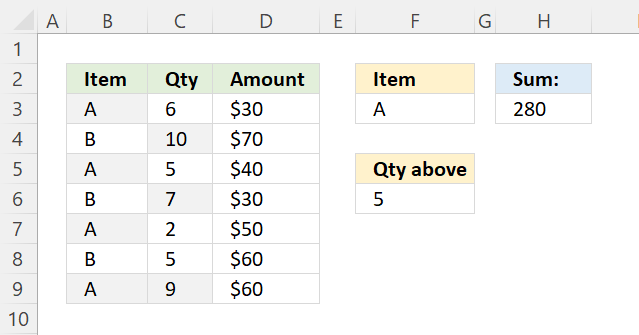The SUMPRODUCT function in cell H3 adds numbers from D3:D9 if the corresponding cell in B3:B9 equals the value in cell F3 or C3:C9 is above the value specified in cell F6.

Formula in cell H3:

=SUMPRODUCT((((B3:B9=F3)+(C3:C9>F6))>0)*D3:D9)

### 5.1 Explaining formula

#### Step 1 - Evaluate condition

The equal sign compares value to value, however, not considering upper and lower cases. You need the EXACT function to do that.

B3:B9=F3

becomes

{"A"; "B"; "A"; "B"; "A"; "B"; "A"}="A"

and returns

{TRUE; FALSE; TRUE; FALSE; TRUE; FALSE; TRUE}.

#### Step 2 - Check if the number is larger than the condition

The larger than sign compares C3:C9 to number in F6, the result is a boolean value TRUE or FALSE.

C3:C9>F6

becomes

{6; 10; 5; 7; 2; 5; 9}>5

and returns

{TRUE; TRUE; FALSE; TRUE; FALSE; FALSE; TRUE}.

#### Step 3 - Perform OR logic between arrays

The plus sign adds value to value, this applies OR logic between the arrays.

TRUE + TRUE = 1
TRUE + FALSE = 1
FALSE + TRUE = 1
FALSE + FALSE = 0 (zero)

This means that at least one condition must be met.

(B3:B9=F3) + (C3:C9>F6)

becomes

{TRUE; FALSE; TRUE; FALSE; TRUE; FALSE; TRUE} + {TRUE; TRUE; FALSE; TRUE; FALSE; FALSE; TRUE}

equals {2; 1; 1; 1; 1; 0; 2}.

In Excel, any other number than 0 (zero) is considered to be TRUE even negative numbers. FALSE is 0 (zero).

#### Step 4 - Check if values in array are larger than 0 (zero)

((B3:B9=F3)+(C3:C9>F6))>0

becomes

{2; 1; 1; 1; 1; 0; 2}>0

and returns {TRUE; TRUE; TRUE; TRUE; TRUE; FALSE; TRUE}.

#### Step 5 - Multiply with numbers

(((B3:B9=F3)+(C3:C9>F6))>0)*D3:D9

becomes

{TRUE; TRUE; TRUE; TRUE; TRUE; FALSE; TRUE}*D3:D9

and returns

{30; 70; 40; 30; 50; 0; 60}.

#### Step 6 - Sum numbers in array

SUMPRODUCT((((B3:B9=F3)+(C3:C9>F6))>0)*D3:D9)

becomes

SUMPRODUCT({30; 70; 40; 30; 50; 0; 60})

and returns 280 in cell H3.

## Get Excel *.xlsx file

### Get the Excel fileSUMPRODUCT-IF-functionv2.xlsx